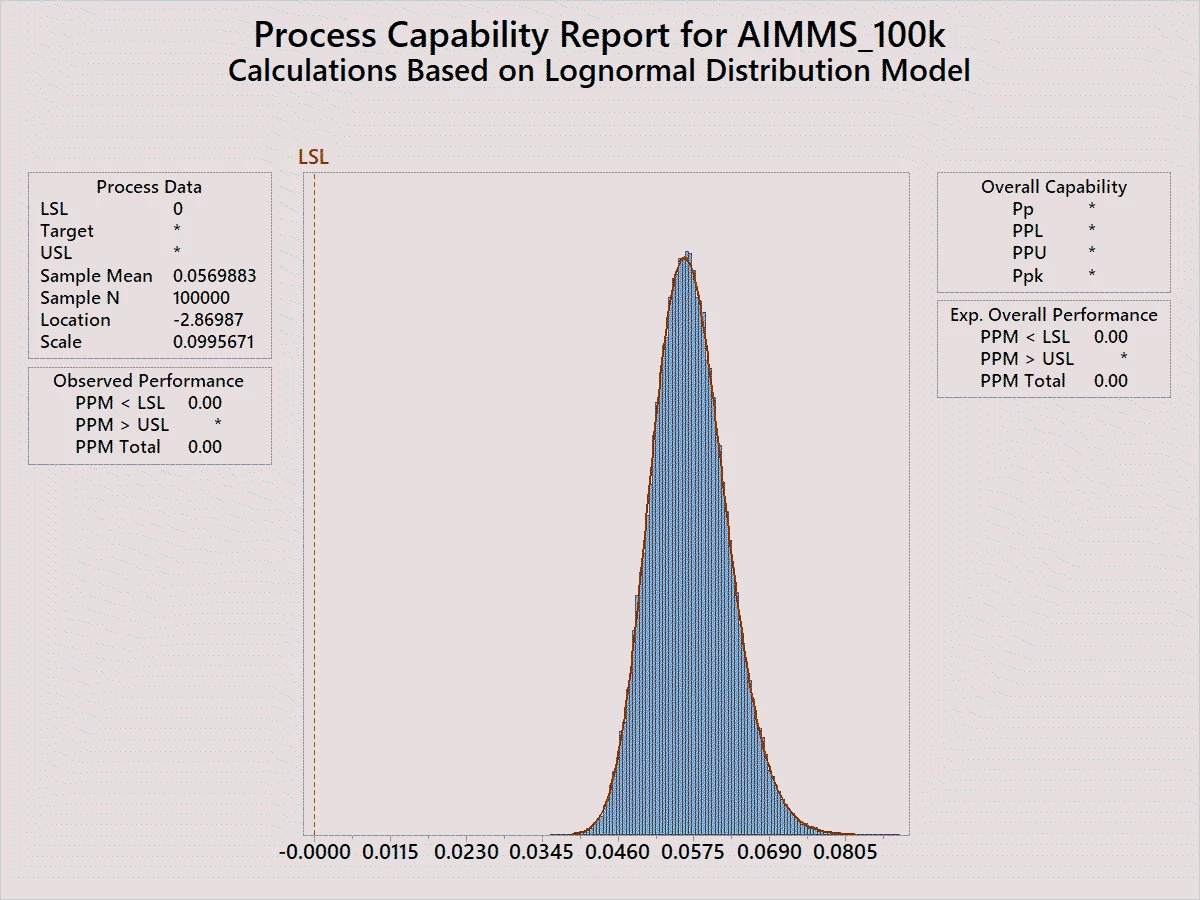# implementation of a lognormal distribution

• 3 replies
• 134 views

• Enthusiast
• 4 replies
I would like to implement a lognormal distribution in AIMMS. I have the parameters mu and sigma as defined on Wikipedia. https://en.wikipedia.org/wiki/Log-normal_distribution. How do I implement these into AIMMS to get the same pdf. I tried to understand the formula in the AIMMS_ref.pdf documentation but implementation of the transformation remains unclear.

### 3 replies

Userlevel 4+3
As what is described in the AIMMS function reference, the function LogNormal( Shape,Lowerbound, Scale ) takes three input parameters, where

Shape = sigma/mu,
Lowerbound = 0
Scale = mu
Userlevel 6+6
Hi @Jan Willem ter Weeme - did you find the information you needed? Do you mind to share how it worked out? (in case others have a similar issue)
Hi @deannezhang, @deannezhang. Thanks for getting in contact. In the end it turned out the implementation needed to be a bit different. The request was to implement:With a mean value of 0.057 and a sigma of 0.1. In the end I implemented simply
lognormal(sigma,0,mean)
which in Minitab yielded exactly the distribution I needed (see mean and scale)So the division of the shape by mu was not needed.
Remains that to my opinion the explanation in the manual is quite hard to follow.